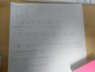# Thermodynamics - How to find an "adiabat"?

• Javier0289

#### Javier0289

Homework Statement
Hi, I've been struggling to find the form of the adiabat of this problem:
Given the fundamental relation, find the form of the adiabats in the P-V plane
(problem 2.3-5. Thermodynamics and an Introduction to Thermostatistics, Callen, pp 42)
Relevant Equations
$${S \over R} = {UV \over N} - {N^3 \over UV}$$
I tried this... but I'm not sure if I'm doing it right or maybe there's a simpler way. Thanks for your time or help :)

The fundamental relation is:

$${S \over R} = {UV \over N} - {N^3 \over UV}$$

but I used $$s=S/N, u=U/N, v=V/N$$ to obtain

$${s \over R} = uv - {1 \over uv}$$

then I did some algebra...

$$u^2 - ({s \over Rv})u - {1 \over v^2} = 0$$

$$u = ({1 \over 2Rv})({s \pm \sqrt{s^2+4R^2}})$$

next I derived the previous expression

$$(\frac{\partial u}{\partial v})_s = -P$$

$$P = ({1 \over 2Rv^2})({s \pm \sqrt{s^2+4R^2}})$$

And my doubt is... how do I get rid of s?

I also tried

$$(\frac{\partial s}{\partial v})_u = {P \over T}$$

and got

$${P \over T} = R(u+{1 \over uv^2})$$

but then how do I get rid of u?

#### Attachments

•WhatsApp Image 2019-09-23 at 18.42.37.jpeg
47.6 KB · Views: 168
Last edited:

You need the answers from the preceding sub-questions. What did you get?

•Javier0289
Hi! from a) all the equations of state are homogeneous of zero order
$${1 \over T} = R({V \over N} + {N^3 \over U^2V})$$
$${P \over T} = R({U \over N} + {N^3 \over UV^2})$$
$${μ \over T} = R({UV \over N^2} + {3N^2 \over UV})$$
b) the temperature is always positive
c) the mechanical equation of state P(T,v)
$$P(T,v) = {RT \over v}[({1 \over vRT}-1)^{-1 \over 2}+({1 \over vRT}-1)^{1 \over 2}]$$
But then I realized that in the last equation is not guaranteed that the entropy will be kept constant, or is it?

You need the answers from the preceding sub-questions. What did you get?
Hi, I put the other results below